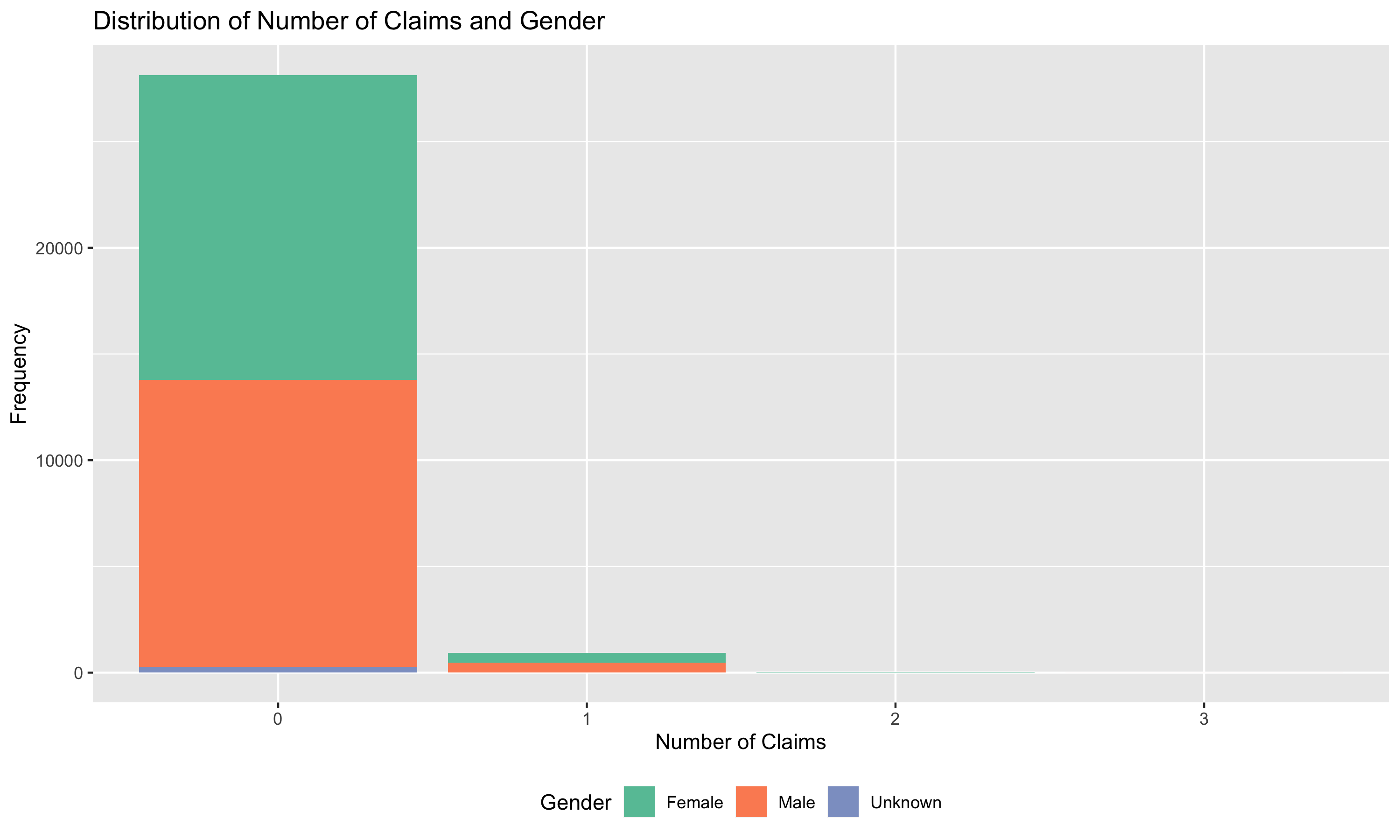Jean-Philippe Boucher, Université du Québec À Montréal (🐦 @J_P_Boucher)

Arthur Charpentier, Université du Québec À Montréal (🐦 @freakonometrics)

Ewen Gallic, Aix-Marseille Université (🐦 @3wen)

# 1 Duration Models

## 1.1 Composition of the portfolio

Analyse the composition of the portfolio: covariates, frequency by covariates.

First, load the training and testing sets, located in the following folder: `data/canada_panel/` (`CanadaPanelTrain.csv` and `CanadaPanelTest.csv`, respectively).

``````library(tidyverse)

Define a function that computes summary statistics for a vector of numerics:

• Average
• Standard deviation
• Min and Max
• Median
• Other percentiles (e.g., 10th, 25th, 75th, and 90th)

Try to account for possible `NA` values. Name the function `my_summary`.

``````#' my_summary
#' Returns a tibble with summary statistics for a numerical vector
#' @param x vector of numerics
my_summary <- function(x) {
tibble(
Average = mean(x, na.rm=TRUE),
Min = min(x, na.rm = TRUE),
Max = max(x, na.rm = TRUE),
`10th percentile` = quantile(x, probs = .1, names = FALSE),
`25th percentile` = quantile(x, probs = .25, names = FALSE),
Median = median(x, na.rm = TRUE),
`75th percentile` = quantile(x, probs = .75, names = FALSE),
`90th percentile` = quantile(x, probs = .9, names = FALSE)
)
}``````

Use the function on a single variable from the training sample:

``my_summary(canada_train\$RA_EXPOSURE_TIME)``

Apply this function to multiple variables of your choice to explore the dataset and put the results in a table. You may either use a for loop, `lapply()` or `map()` (pick the option you are more comfortable with). Do not forget to add the variable name to be able to identify the variables.

``````some_variables <- c("RA_DISTANCE_DRIVEN", "RA_NBTRIP", "RA_HOURS_DRIVEN")

# Using a for loop
for( var in some_variables ) {
bind_rows(
)
}

# Using lapply
bind_rows()

# Using map
dplyr::select(!!some_variables) %>%
purrr::map(my_summary) %>%
bind_rows(.id = "variable")

Plot the distribution of claims on a barplot. You may display the distribution of gender among each claims levels (by showing the proportion of each gender within the bars). The result may look like the figure displayed below:``````p_distribution_claims_gender <-
dplyr::group_by(RA_ACCIDENT_IND, RA_GENDER) %>%
dplyr::tally() %>%
mutate(RA_GENDER = factor(RA_GENDER, levels = c("2", "1", "3"),
labels = c("Female", "Male", "Unknown"))) %>%
ggplot(data = ., aes(x = reorder(RA_ACCIDENT_IND, -n), y = n, fill = RA_GENDER)) +
geom_col() +
scale_fill_manual(name = "Gender",
values = c("Female" = "#66c2a5",
"Male" = "#fc8d62",
"Unknown" = "#8da0cb")) +
labs(x = "Number of Claims", y = "Frequency",
title = "Distribution of Number of Claims and Gender") +
# Legend below the graph
theme(legend.position = "bottom")

ggsave(p_distribution_claims_gender,
file = "figs/p_distribution_claims_gender.png", width = 10, height = 6)

p_distribution_claims_gender``````

Plot a boxplot of exposure time depending on marital status and vehicle use. Use faceting to separate the data according to vehicule use. The resulting plot should look like the one below: